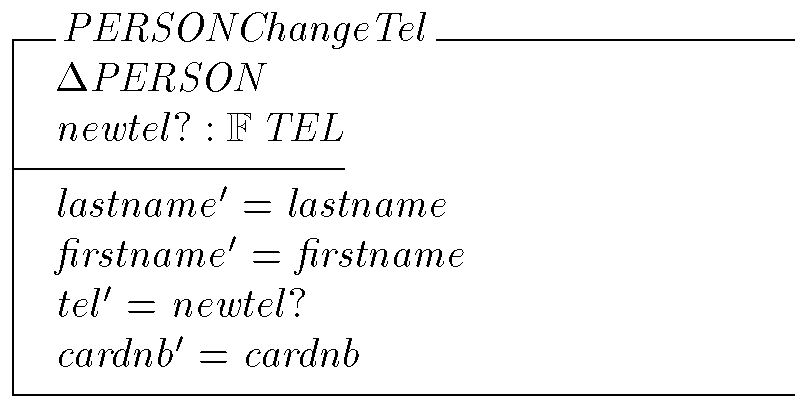Theorems Generation

RoZ can also generate a theorem to validate proposed pre-conditions for each operation. It uses the information given in the operation form. Let us consider the operation "ChangeTel" which changes the value of the "tel" attribute. The automatically generated specification for this operation is :Its actual pre-condition can be computed from the text of the specification and the constraints listed in the included schemas. When specifications get complex, it is good software engineering practice to state these pre-conditions more explicitly. For the "ChangeTel" operation, we identify the pre-condition : the new set of telephone numbers must not be empty.

In fact, we guess that this pre-condition is strong enough to imply the actual pre-condition of the operation. This predicate states that the new set of telephone numbers must not be empty. We add it to the field "Pre Conditions" of the operation "ChangeTel":

newtel? \neq \empty

Then RoZ generates the following theorem is generated for "ChangeTel":

\begin{theorem}{ PERSONChangeTel \_Pre}
\forall PERSON ;newtel? : \finset TEL |
newtel? \neq \empty @
\pre PERSONChangeTel
\end{theorem}

It means that the proposed pre-condition (newtel? \neq \empty ) is stronger than the computed pre-condition (pre PERSONChangeTel ).

If you want to prove the theorems generated, you can use the Z-EVES theorem prover. For instance, the pre-condition theorem for "ChangeTel" is proved automatically using the following commands :

try lemma PERSONChangeTel\_Pre;
prove by reduce;

which demonstrates that the proposed pre-condition is correct.

last update by Sophie Dupuy, 14 october 1999